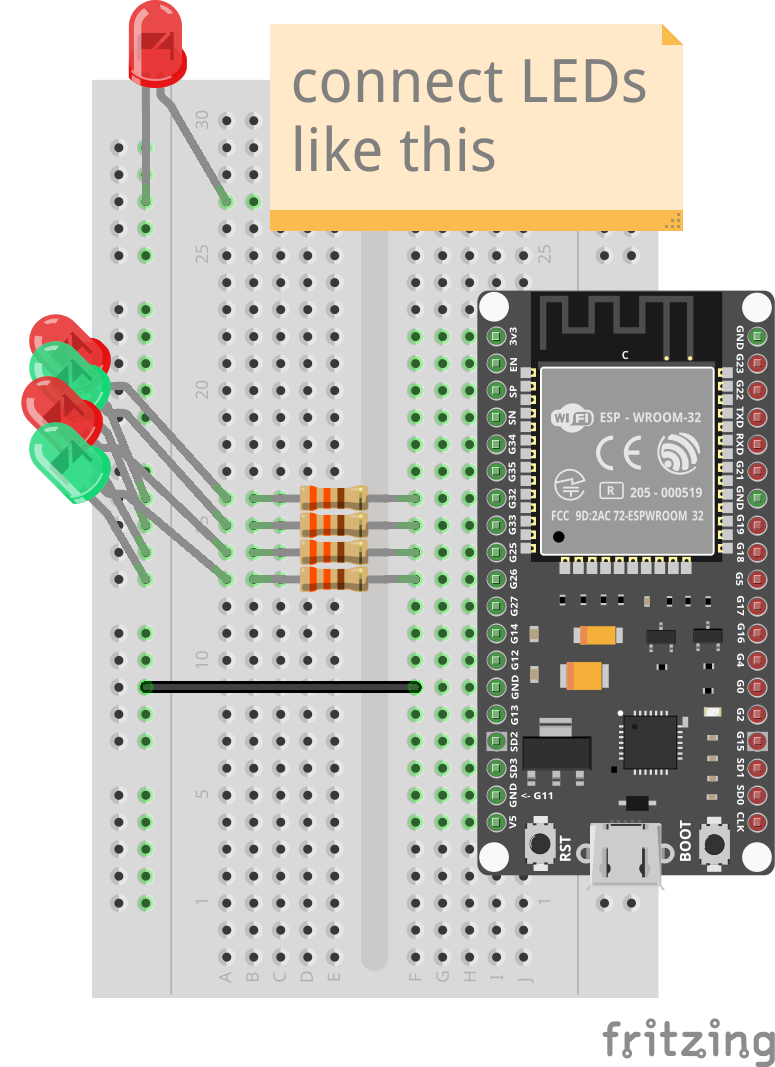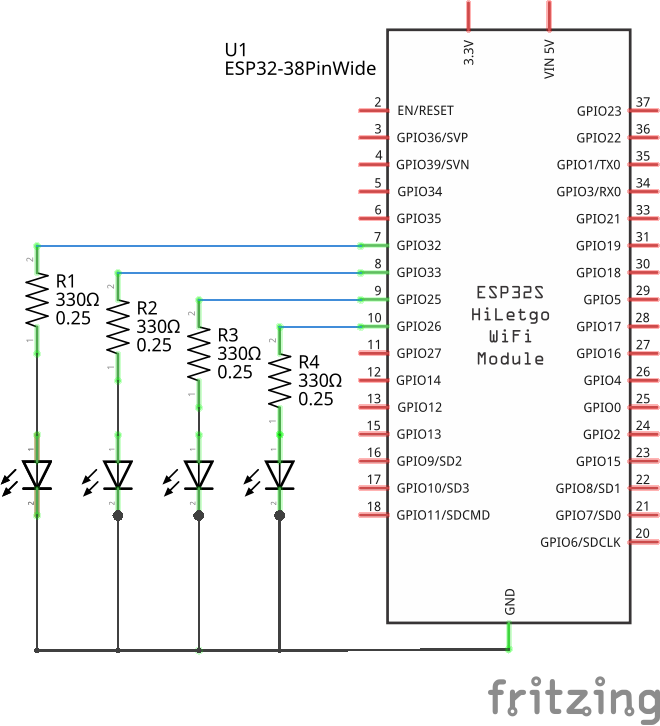# Running LEDs

In this tutorial we will connect multiple LEDs to the ESP32 and control them independently. We will also see how to use a `List` to simplify the code.

## Prerequisites

This tutorial assumes that you know how to control a single LED. If necessary, have a look at the single LED tutorial first.

## Setup

Connect 4 LEDs, each with a current-limiting resistor, to pins 32, 33, 25, and 26.

As for the single LED case, remember that the long leg of the LED must be on the positive side (towards the pins of the ESP32).## Code

We can control each LED by setting the corresponding pin to low or high.

You can modify the `led.toit` from the single-LED tutorial and change the pin number. The new LEDs should behave exactly the same as before. The only difference is that they are connected to different pins.

Let's use all LEDs at the same time. For this, create a new file and save it as `running_led.toit`.

You could already start Jaguar to `jag watch running_led.toit` so you can immediately see the changes you make to the file.

Enter the following code into the file.

```import gpio

main:
leds := [
gpio.Pin 32 --output,
gpio.Pin 33 --output,
gpio.Pin 25 --output,
gpio.Pin 26 --output,
]

// Turn each LED on for 500ms.
leds.do:
it.set 1
sleep --ms=500
it.set 0

// Shut down each pin.
leds.do: it.close```

The `leds.do` is a method on `List` that takes a block ("code") as argument and calls it for every element in the list. Here there are four elements in the list, and the block is thus called four times. Each time the code is executed with it being set to the corresponding element. The first time the block is called, it is thus equal to pin 32. The next time it is equal to pin 33, ...

This is a good start, but we would like to see the LEDs cycle continuously. Let's change the program to continue running through the LEDs in an infinite loop:

```import gpio

main:
leds := [
gpio.Pin 32 --output,
gpio.Pin 33 --output,
gpio.Pin 25 --output,
gpio.Pin 26 --output,
]

while true:
// Turn each LED on for 200ms.
leds.do:
it.set 1
sleep --ms=200
it.set 0```

Note that you could also use an index variable to achieve the same behavior:

```import gpio

main:
leds := [
gpio.Pin 32 --output,
gpio.Pin 33 --output,
gpio.Pin 25 --output,
gpio.Pin 26 --output,
]

i := 0
while true:
// Take the next led and turn it on for 200ms.
led := leds[i]
led.set 1
sleep --ms=200
led.set 0
// Increment the index, wrapping around when we reach the
// end of the list.
i = (i + 1) % leds.size```

## Exercises

As long as the connections were done correctly you can't damage your hardware by changing your program. If you change the connections, make sure that LEDs are always in series with a resistor.

• Change the speed of the cycling.
• Instead of turning the cycling LED on, have all LEDs turned on at the start and then turn off one LED at a time.
• Instead of cycling the LED, let it move from left to right and back. A bit like the K.I.T.T lights from the Knight Rider series.